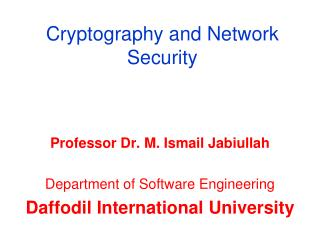DownloadDownload PresentationCryptography and Network Security

# Cryptography and Network Security

Download Presentation## Cryptography and Network Security

- - - - - - - - - - - - - - - - - - - - - - - - - - - E N D - - - - - - - - - - - - - - - - - - - - - - - - - - -
##### Presentation Transcript

1. Cryptography and Network Security Professor Dr. M. Ismail Jabiullah Department of Software Engineering Daffodil International University

2. Topics • Cryptography • Cryptographic Terms • Cryptographic Components • Encryption/Decryption • Types of Cryptographic Algorithms • Symmetric Key Cryptography • Caesar Cipher • Concepts • Problems

3. 0 1 2 3 4 5 6 7 8 9 10 11 12 A B C D E F G H I J K L M 13 14 15 16 17 18 19 20 21 22 23 24 25 N O P Q R S T U V W X Y Z Text space for writing documents

4. Plaintext in KKey Algorithm Ciphertext in Ciphertext in Key Algorithm Plaintext in Text Number Number Text ~ Text Number Number Text C 2 K = 4 6 G G 6 K = 4 2 C O 14 18 S S 18 14 O M 12 Forward 16 Q Q 16 Backward 12 M P 15 19 T T 19 15 P U 20 24 Y Y 24 20 U T 19 23 X X 23 19 T E 4 8 I I 8 4 E R 17 21 V V 21 17 R Caesar Cipher Ciphertext : GSQTYXIV Plaintext : COMPUTER

5. Caesar Cipher Perform the Encryption/decryption operation with key value 5 COME HERE PAY MORE MONEY

6. Caesar Cipher • Julius Caesar • Process: • Replace each letter of the alphabet with letter standing three places further down the alphabet. • The alphabet is wrapped around, so that the letter following Z is A. • Advantages: • Simply try all possible 25 ways. • Key values are within 25. • Characteristics • The encryption and decryption algorithms are known. • There are only 25 keys to try. • The language of the plaintext is known and easily recognizable.

7. Caesar Cipher

8. Cryptography Components • Plaintext • Sender • Receiver • Encryption Algorithm • Ciphertext • Decryption Algorithm

9. Key Elements Plaintext = P Encryption Algorithm = E Decryption Algorithm = D Ciphertext = C Key = K

10. Symmetric-key Cryptography Or Single Key Cryptography Private Key Cryptography

11. Public-key Cryptography Public-key algorithms are more efficient for short messages.

12. Signing the Whole Document

13. Cryptography Algorithms in the world are in two types: • Symmetric-key Cryptography Algorithms or • Secret key Cryptography Algorithms or • Single key Cryptography Algorithms • Asymmetric-key Cryptography Algorithms or • Public key Cryptography Algorithms or • Public-Private key Cryptography Algorithms

14. Problems • Perform Encryption and Decryption for at least 3 plaintext with key values more than 15.

15. That’s All For Today